# Introduction¶

The most basic features of galpy are its ability to display rotation curves and perform orbit integration for arbitrary combinations of potentials. This section introduce the most basic features of galpy.potential and galpy.orbit.

## Rotation curves¶

The following code example shows how to initialize a Miyamoto-Nagai disk potential and plot its rotation curve

>>> from galpy.potential import MiyamotoNagaiPotential
>>> mp= MiyamotoNagaiPotential(a=0.5,b=0.0375,normalize=1.)
>>> mp.plotRotcurve(Rrange=[0.01,10.],grid=1001)


The normalize=1. option normalizes the potential such that the radial force is a fraction normalize=1. of the radial force necessary to make the circular velocity 1 at R=1.

Similarly we can initialize other potentials and plot the combined rotation curve

>>> from galpy.potential import NFWPotential, HernquistPotential
>>> mp= MiyamotoNagaiPotential(a=0.5,b=0.0375,normalize=.6)
>>> np= NFWPotential(a=4.5,normalize=.35)
>>> hp= HernquistPotential(a=0.6/8,normalize=0.05)
>>> from galpy.potential import plotRotcurve
>>> plotRotcurve([hp,mp,np],Rrange=[0.01,10.],grid=1001,yrange=[0.,1.2])


Note that the normalize values add up to 1. such that the circular velocity will be 1 at R=1. The resulting rotation curve is approximately flat. To show the rotation curves of the three components do

>>> mp.plotRotcurve(Rrange=[0.01,10.],grid=1001,overplot=True)
>>> hp.plotRotcurve(Rrange=[0.01,10.],grid=1001,overplot=True)
>>> np.plotRotcurve(Rrange=[0.01,10.],grid=1001,overplot=True)


You’ll see the following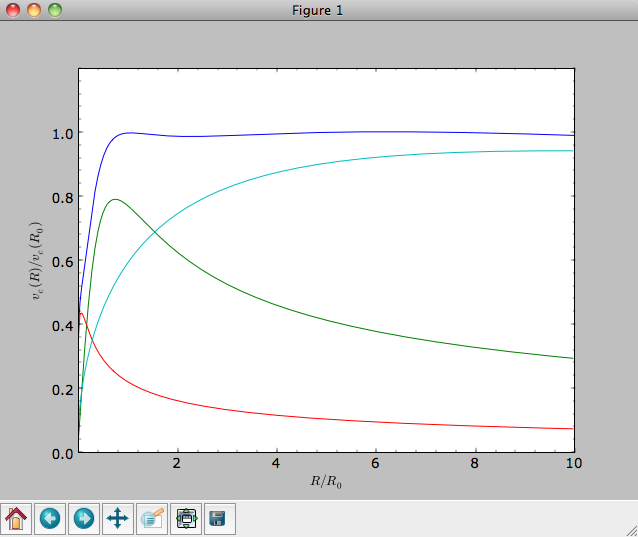As a shortcut the [hp,mp,np] Milky-Way-like potential is defined as

>>> from galpy.potential import MWPotential


This is not the recommended Milky-Way-like potential in galpy. The (currently) recommended Milky-Way-like potential is MWPotential2014:

>>> from galpy.potential import MWPotential2014


MWPotential2014 has a more realistic bulge model and is actually fit to various dynamical constraints on the Milky Way (see here and the galpy paper).

## Units in galpy¶

Above we normalized the potentials such that they give a circular velocity of 1 at R=1. These are the standard galpy units (sometimes referred to as natural units in the documentation). galpy will work most robustly when using these natural units. When using galpy to model a real galaxy with, say, a circular velocity of 220 km/s at R=8 kpc, all of the velocities should be scaled as v= V/[220 km/s] and all of the positions should be scaled as x = X/[8 kpc] when using galpy’s natural units.

For convenience, a utility module bovy_conversion is included in galpy that helps in converting between physical units and natural units for various quantities. For example, in natural units the orbital time of a circular orbit at R=1 is $$2\pi$$; in physical units this corresponds to

>>> from galpy.util import bovy_conversion
>>> print 2.*numpy.pi*bovy_conversion.time_in_Gyr(220.,8.)
0.223405444283


or about 223 Myr. We can also express forces in various physical units. For example, for the Milky-Way-like potential defined in galpy, we have that the vertical force at 1.1 kpc is

>>> from galpy.potential import MWPotential2014, evaluatezforces
>>> -evaluatezforces(1.,1.1/8.,MWPotential2014)*bovy_conversion.force_in_pcMyr2(220.,8.)
2.0259181908629933


which we can also express as an equivalent surface-density by dividing by $$2\pi G$$

>>> -evaluatezforces(1.,1.1/8.,MWPotential2014)*bovy_conversion.force_in_2piGmsolpc2(220.,8.)
71.658016957792356


Because the vertical force at the solar circle in the Milky Way at 1.1 kpc above the plane is approximately $$70\,(2\pi G\, M_\odot\,\mathrm{pc}^{-2})$$ (e.g., 2013arXiv1309.0809B), this shows that our Milky-Way-like potential has a realistic disk (at least in this respect).

bovy_conversion further has functions to convert densities, masses, surface densities, and frequencies to physical units (actions are considered to be too obvious to be included); see here for a full list. As a final example, the local dark matter density in the Milky-Way-like potential is given by

>>> MWPotential2014.dens(1.,0.)*bovy_conversion.dens_in_msolpc3(220.,8.)
0.0075419566970079373


or

>>> MWPotential2014.dens(1.,0.)*bovy_conversion.dens_in_gevcc(220.,8.)
0.28643101789044584


or about $$0.0075\,M_\odot\,\mathrm{pc}^{-3} \approx 0.3\,\mathrm{GeV\,cm}^{-3}$$, in line with current measurements (e.g., 2012ApJ...756...89B).

When galpy Orbits are initialized using a distance scale ro= and a velocity scale vo= output quantities returned and plotted in physical coordinates. Specifically, positions are are returned in units of kpc, velocities in km/s, energies and the Jacobi integral in (km/s)^2, the angular momentum o.L() and actions in km/s kpc, frequencies in 1/Gyr, and times and periods in Gyr.

## Orbit integration¶

We can also integrate orbits in all galpy potentials. Going back to a simple Miyamoto-Nagai potential, we initialize an orbit as follows

>>> from galpy.orbit import Orbit
>>> mp= MiyamotoNagaiPotential(a=0.5,b=0.0375,amp=1.,normalize=1.)
>>> o= Orbit(vxvv=[1.,0.1,1.1,0.,0.1])


Since we gave Orbit() a five-dimensional initial condition [R,vR,vT,z,vz], we assume we are dealing with a three-dimensional axisymmetric potential in which we do not wish to track the azimuth. We then integrate the orbit for a set of times ts

>>> import numpy
>>> ts= numpy.linspace(0,100,10000)
>>> o.integrate(ts,mp,nethod='odeint')


Now we plot the resulting orbit as

>>> o.plot()


Which gives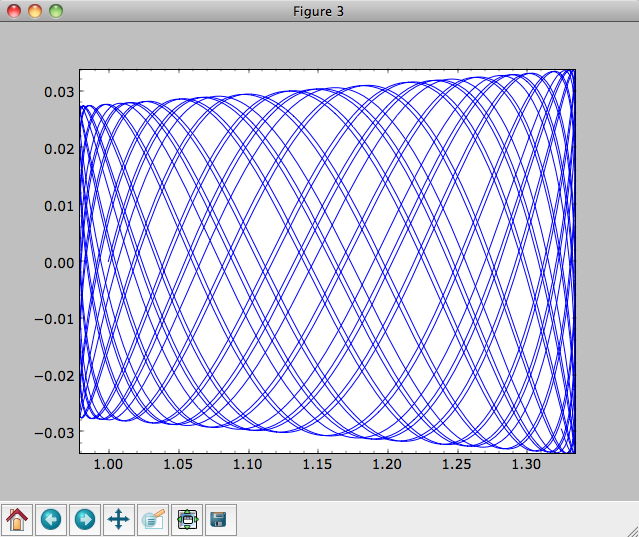The integrator used is not symplectic, so the energy error grows with time, but is small nonetheless

>>> o.plotE(normed=True)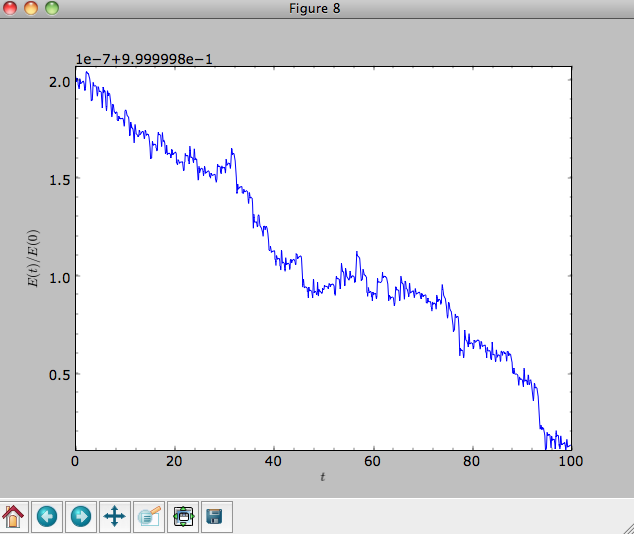When we use a symplectic leapfrog integrator, we see that the energy error remains constant

>>> o.integrate(ts,mp,method='leapfrog')
>>> o.plotE(xlabel=r'$t$',ylabel=r'$E(t)/E(0)$')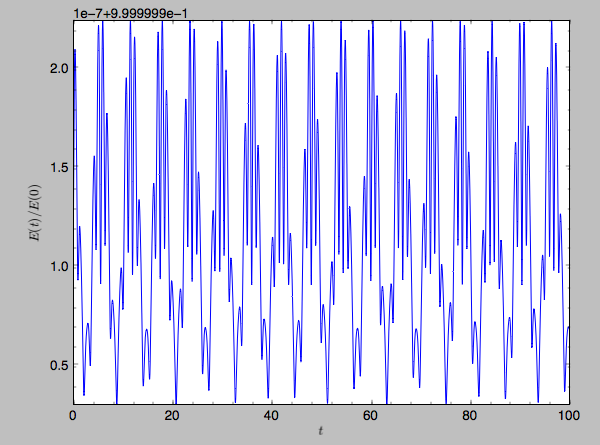Because stars have typically only orbited the center of their galaxy tens of times, using symplectic integrators is mostly unnecessary (compared to planetary systems which orbits millions or billions of times). galpy contains fast integrators written in C, which can be accessed through the method= keyword (e.g., integrate(...,method='dopr54_c') is a fast high-order Dormand-Prince method).

When we integrate for much longer we see how the orbit fills up a torus (this could take a minute)

>>> ts= numpy.linspace(0,1000,10000)
>>> o.integrate(ts,mp,method='odeint')
>>> o.plot()As before, we can also integrate orbits in combinations of potentials. Assuming mp, np, and hp were defined as above, we can

>>> ts= numpy.linspace(0,100,10000)
>>> o.integrate(ts,[mp,hp,np])
>>> o.plot()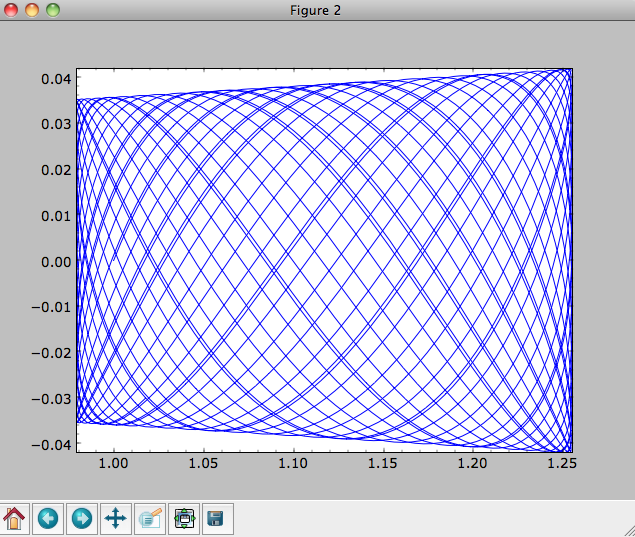Energy is again approximately conserved

>>> o.plotE(xlabel=r'$t$',ylabel=r'$E(t)/E(0)$')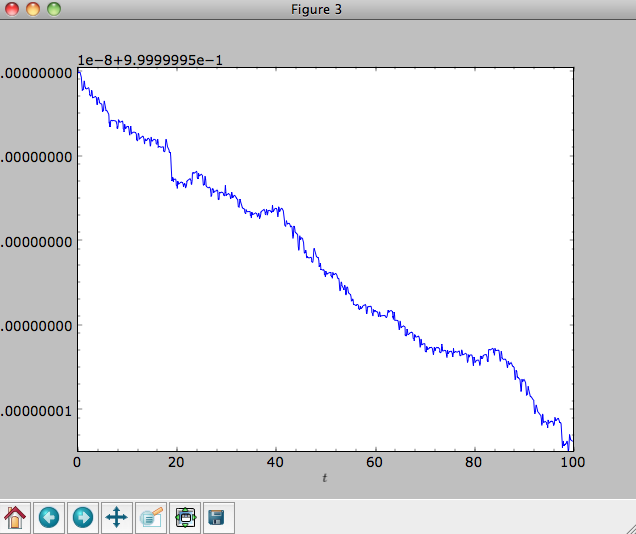## Escape velocity curves¶

Just like we can plot the rotation curve for a potential or a combination of potentials, we can plot the escape velocity curve. For example, the escape velocity curve for the Miyamoto-Nagai disk defined above

>>> mp.plotEscapecurve(Rrange=[0.01,10.],grid=1001)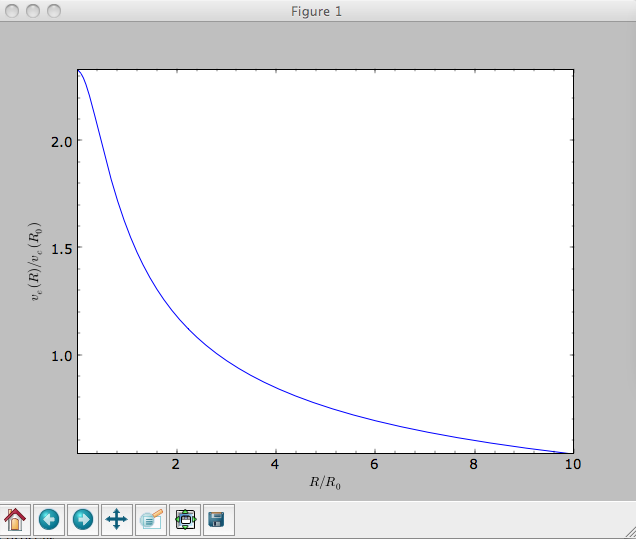or of the combination of potentials defined above

>>> from galpy.potential import plotEscapecurve
>>> plotEscapecurve([mp,hp,np],Rrange=[0.01,10.],grid=1001)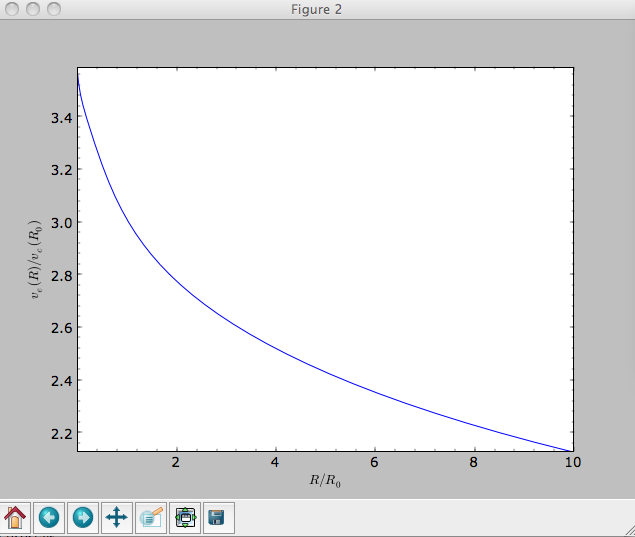For the Milky-Way-like potential MWPotential2014, the escape-velocity curve is

>>> plotEscapecurve(MWPotential2014,Rrange=[0.01,10.],grid=1001)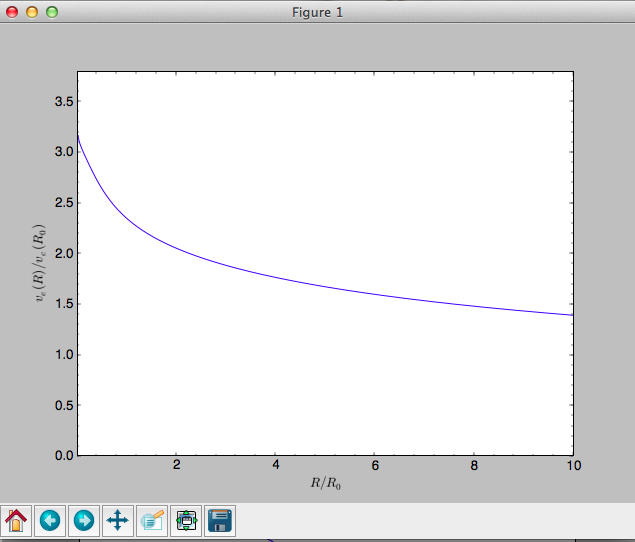At the solar radius, the escape velocity is

>>> from galpy.potential import vesc
>>> vesc(MWPotential2014,1.)
2.3316389848832784


Or, for a local circular velocity of 220 km/s

>>> vesc(MWPotential2014,1.)*220.
512.96057667432126


similar to direct measurements of this (e.g., 2007MNRAS.379..755S and 2014A%26A...562A..91P).